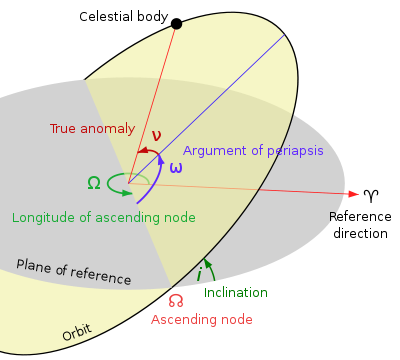# Orbital node

﻿
Orbital nodeThe ascending node.

An orbital node is one of the two points where an orbit crosses a plane of reference to which it is inclined. An orbit which is contained in the plane of reference (called non-inclined) has no nodes.

## Planes of reference

Common planes of reference include:

## Node distinction

If a reference direction from one side of the plane of reference to the other is defined, the two nodes can be distinguished. For geocentric and heliocentric orbits, the ascending node (or north node) is where the orbiting object moves north through the plane of reference, and the descending node (or south node) is where it moves south through the plane. In the case of objects outside the Solar System, the ascending node is the node where the orbiting secondary passes away from the observer, and the descending node is the node where it moves towards the observer., p. 137.

The position of the node may be used as one of a set of parameters, called orbital elements, which describe the orbit. This is done by specifying the longitude of the ascending node (or, sometimes, the longitude of the node.)

The line of nodes is the intersection of the object's orbital plane with the plane of reference. It passes through the two nodes.

## Symbols and nomenclature

The symbol of the ascending node is(Unicode: U+260A, ☊), and the symbol of the descending node is(Unicode: U+260B, ☋). In medieval and early modern times the ascending and descending nodes were called the dragon's head (Latin: caput draconis, Arabic: ra's al-jauzahar) and dragon's tail (Latin: cauda draconis), respectively., p. 141; , p. 245. These terms originally referred to the times when the moon crossed the apparent path of the sun in the sky. Also, corruptions of the Arabic term such as ganzaar, genzahar, geuzaar and zeuzahar were used in the medieval West to denote either of the nodes., pp. 196–197; , p. 65; , pp. 95–96. The Greek terms αναβιβάζων and καταβιβάζων were also used for the ascending and descending nodes, giving rise to the English words anabibazon and catabibazon., ¶27.

## Earth orbit nodes

For the orbit of the Earth around the Sun is important the line of nodes formed by the ecliptic and the equator. There are two special days in which this line points to the sun. For these days, night and day take the same time. These two points in the sun orbit are named equinoxes.

## Lunar nodes

For the orbit of the Moon around the Earth, the reference plane is taken to be the ecliptic, not the equatorial plane. The gravitational pull of the Sun upon the Moon causes its nodes, called the lunar nodes, to precess gradually westward, performing a complete circle in approximately 18.6 years.

Wikimedia Foundation. 2010.

### Look at other dictionaries:

• Node — In general, a node is a localised swelling (a knot ) or a point of intersection (a vertex). Node may refer to: In mathematics Node (autonomous system), behaviour for an ordinary differential equation near a critical point Node (graph theory), a… …   Wikipedia

• Orbital elements — are the parameters required to uniquely identify a specific orbit. In celestial mechanics these elements are generally considered in classical two body systems, where a Kepler orbit is used (derived from Newton s laws of motion and Newton s law… …   Wikipedia

• Node Module — redirects here. For other uses, see Node Module (disambiguation). The Node Module (NM) is a pressurized module of the Russian Segment (RS or ROS) of the International Space Station (ISS) and will be used in the OPSEK space station. NM is being… …   Wikipedia

• Orbital inclination change — is an orbital maneuver aimed at changing the inclination of an orbiting body s orbit. This maneuver is also known as an orbital plane change as the plane of the orbit is tipped. This maneuver requires a change in the orbital velocity vector… …   Wikipedia

• Orbital perturbation analysis (spacecraft) — Isaac Newton in his Philosophiæ Naturalis Principia Mathematica demonstrated that the gravitational force between two mass points is inversely proportional to the square of the distance between the points and fully solved corresponding two body… …   Wikipedia

• Orbital mechanics — A satellite orbiting the earth has a tangential velocity and an inward acceleration. Orbital mechanics or astrodynamics is the application of ballistics and celestial mechanics to the practical problems concerning the motion of rockets and other… …   Wikipedia

• Orbital state vectors — In astrodynamics or celestial dynamics orbital state vectors (sometimes state vectors) are vectors of position ( ) and velocity ( ) that together with their time (epoch) ( ) uniquely determine the state of an orbiting body. State vectors are… …   Wikipedia

• Orbital period — For the music album, see Orbital Period (album). The orbital period is the time taken for a given object to make one complete orbit about another object. When mentioned without further qualification in astronomy this refers to the sidereal period …   Wikipedia

• Node 4 — The Node STA in the Space Station Processing Facility during 2010 Node 4, also known as the Docking Hub System (DHS), is a proposed module of the International Space Station (ISS). NASA is considering a 40 month design and development effort for… …   Wikipedia

• Orbital resonance — For the science fiction novel by John Barnes, see Orbital Resonance (novel). In celestial mechanics, an orbital resonance occurs when two orbiting bodies exert a regular, periodic gravitational influence on each other, usually due to their… …   Wikipedia